## How do I solve a system with boundary conditions t...

Hello everyone,

I am having trouble trying to solve a system of differential equations. The modeling was made from the equilibrium equations of a pressure vessel. The set of equations is shown below: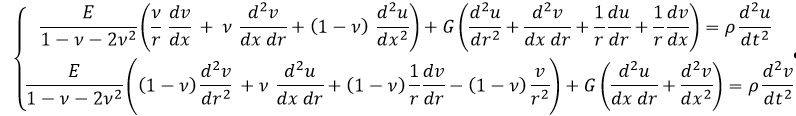As you see it is a set of two second-order partial differential equations. So, we need four boundary conditions. This one is the first. It means that the left end of the pressure vessel is fixed.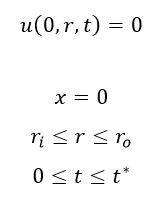This one is the second boundary condition. It means that the right end of the pressure vessel is free.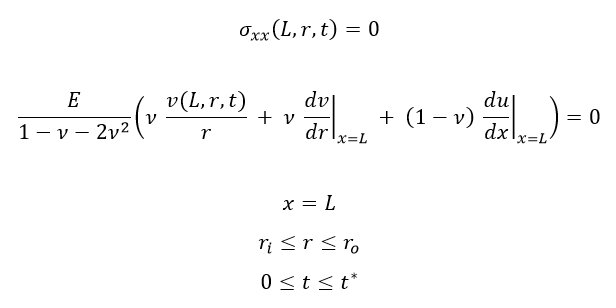This one is the third boundary condition. It means that the inner surface of the pressure vessel is subject to an external load: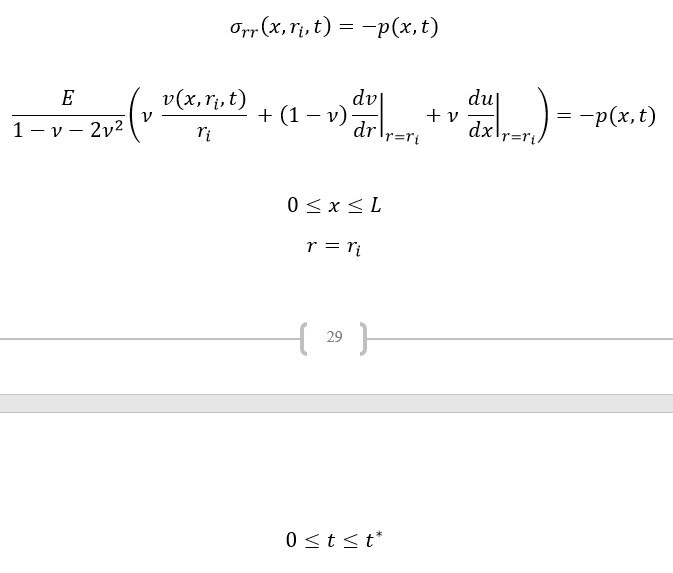At last, we have the fourth boundary condition. It means that the outer surface of the pressure vessel is free.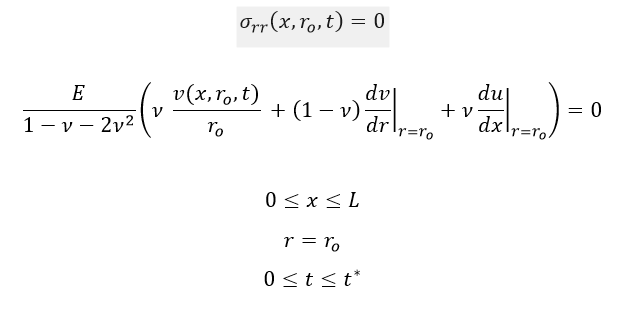The first test I have been trying to do is the static case. In this case, the time terms (the right side of the two equations shown) is zero.

The maple commands that I am using are the following:

```restart; E := 200*10^9; nu := .33; G := E/(2*(1+nu)); RI := 0.254e-1; RO := 2*RI; p := proc (x) options operator, arrow; 50000000 end proc; sys := [E*(nu*(diff(v(x, r), x))/r+nu*(diff(diff(v(x, r), x), r))+(1-nu)*(diff(diff(u(x, r), x), x)))/(-2*nu^2-nu+1)+G*(diff(diff(u(x, r), r), r)+diff(diff(v(x, r), x), r)+(diff(u(x, r), r))/r+(diff(v(x, r), x))/r) = 0, E*((1-nu)*(diff(diff(v(x, r), r), r))+nu*(diff(diff(u(x, r), x), r))+(1-nu)*(diff(v(x, r), r))/r-(1-nu)*v(x, r)/r^2)/(-2*nu^2-nu+1)+G*(diff(diff(u(x, r), r), x)+diff(diff(v(x, r), x), x)) = 0]; BCs := {E*(nu*v(L, r)/r+nu*(D(v))(L, r)+(1-nu)*(D(u))(L, r))/(-2*nu^2-nu+1) = 0, E*(nu*v(x, RI)/RI+(1-nu)*(D(v))(x, RI)+nu*(D(u))(x, RI))/(-2*nu^2-nu+1) = -p(x), E*(nu*v(x, RO)/RO+(1-nu)*(D(v))(x, RO)+nu*(D(u))(x, RO))/(-2*nu^2-nu+1) = 0, u(0, r) = 0}

sol := pdsolve(sys, BCs, numeric)```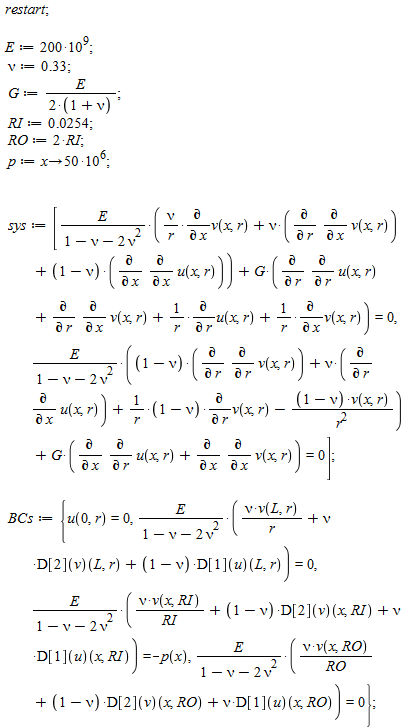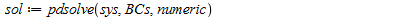I am getting the following error:

Error, (in pdsolve/numeric/process_IBCs) initial/boundary conditions must depend upon exactly one of the independent variables: 0.1459531181e12*v(L, r)/r+0.1459531181e12*(D(v))(L, r)+0.2963290579e12*(D(u))(L, r) = 0

In this case, my boundary conditions do depend on more than one independent variable. How do I proceed?

Pedro Guaraldi

## Putting entries of a matrix into a new matrix but ...

Assume you have a matrix A and somewhere you want to make a copy of it like B and working with them independently. Let's say you have a loop and after doing a proc on B again you have to make it equal to A. So changes on B shouldn't effect on A. What is the common way of taking such copies of A in Maple?

The following methods don't work.

```A:=Matrix(3);
B:=A;
B(1,1):=1;
A;```
```A:=Matrix(3);
B:=subs(B=A,B);
B(1,1):=1;
A;```

What I came up with is the following but it will look weird if one really needs to write something meaningless like *2/2.

```A:=Matrix(3);
B := (1/2)*subs(B = 2*A, B);
B(1,1):=1;
A;```

## Different results between terminal and GUI...

I have the following expression:

expand((((o * (((h * h - h * v) * (a * h - a * v) * h * h * h * h * a * a * h - h * h * h * a * a * h * o * (h * h - h * v) * (a * h - a * v)) * a * a * h * h * h * h * h - h * h * h * a * a * h * h * h * h * h * a * a * h * (a * h - a * o) * (h * h - h * v) * (h - v)) * h * a * a * h * h * h * h * a * a * h * a * a * h * h * h * h * h - h * h * h * a * a * h * h * h * h * h * a * a * h * a * a * h * h * h * h * h * ((v * (a * h - a * v) * h * h * h * a * a * h - h * a * a * h * (h * h - h * v) * v * (a * h - a * v)) * a * a * h * h * h * h * h - h * a * a * h * h * h * h * a * a * h * (a * h - a * o) * (v * h * h * h - h * (h * h - h * v) * v))) * h * a * a * h * h * a * a * h * a * a * h * h - h * h * h * a * a * h * h * h * h * h * a * a * h * a * a * h * h * h * h * h * h * a * a * h * h * h * h * a * a * h * a * a * h * h * h * h * h * o * (((a * h - a * v) * h * a * a * h - a * a * h * o * (a * h - a * v)) * a * a * h * h - a * a * h * h * a * a * h * (a * h - a * o) * (h - v))) * h * h * a * a * h * h * h * h * a * a * h * a * a * h * h * h * h * h - h * h * h * a * a * h * h * h * h * h * a * a * h * a * a * h * h * h * h * h * h * a * a * h * h * h * h * a * a * h * a * a * h * h * h * h * h * h * a * a * h * h * a * a * h * a * a * h * h * (o * (v * (a * h - a * v) * h * h * h * a * a * h - h * a * a * h * (h * h - h * v) * v * (a * h - a * v)) * a * a * h * h * h * h * h - h * a * a * h * h * h * h * a * a * h * (a * h - a * o) * (v * v * h * h * h - h * (h * h - h * v) * v * v))) );

When I tun it through the Maple GUI I get this result:

``````-2*a^23*h^40*o*v^2+2*a^23*h^40*v^3
``````

This is also what Mathematica gives me. However, if I run it through the Maple command line I get this result:

``````-a^77*h^97*o*v^2+a^77*h^97*v^3-a^23*h^40*o*v^2+a^23*h^40*v^3
``````

Why are the results different?

I need a tutor for maple 17. Its very hard to program formulas and all even if its an online tutor im willing to pay

## Solution of PDE...

I am trying to see the solution to a PDE that I am coding with initial and boundary conditions. I know with the ODE, it shows the solution, but with the PDE I cannot seem to see it. Any suggestions?

## DEplot - Error, (in DEtools/DEplot) vars must be d...

Hello. I have a problem with DEplot and I hope someone could help me with this:## Fractional Differential Transform method...

Hi!

I hope every one is ok.

I am running this code (see below)

m := 2;

X := 14;

Y := 18;

a := 1; b := 1; c := .1; d := 1;

alpha := 1;

for k from 0 to m do X[k+1] := GAMMA(k*alpha+1)*(a*X(k)-b*(sum(X(s)*Y(k-s), s = 0 .. k)))/GAMMA(k*alpha+1+1); Y[k+1] := GAMMA(k*alpha+1)*(-c*Y(k)+d*(sum(X(s)*Y(k-s), s = 0 .. k)))/GAMMA(k*alpha+1+1) end do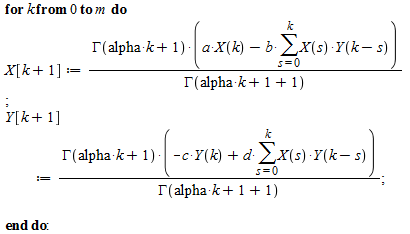x := 0; y := 0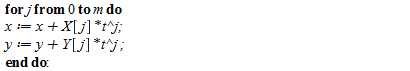The following message pop out.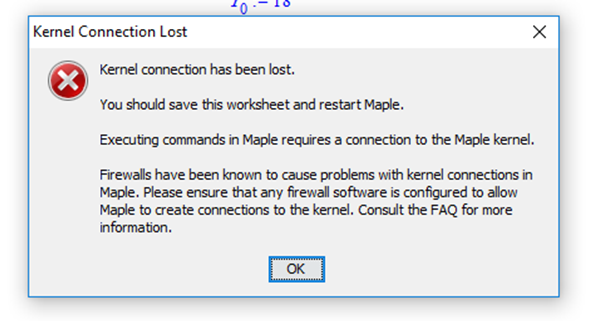\

## How would i start to write a procedure to decrypt ...

Suppose i have a message X encrypted using RSA with public code (n,e) and ascii (Here we treat the message as an array so don't worry about block-length) how would one go about writing a procedure that given a public key (n,e) with small n and a ciphertext message x will decrypt x. I have been looking online to see how we can get maple to take ascii into consideration but to no avail any help would be appreciated

## Elementwise matrix multiplication fails. Why?...

Consider the following code:

```LM := [
Matrix([[1,2],[3,4]]),
Matrix([[5,6],[7,8]])
];
A := Matrix([[0,1],[1,0]]);
map(x -> A . x,LM);
A .~ LM;```

where LM is a list of two matrices (just a test example), and A is some (test)matrix that I want to multiply onto each of these two matrices from the left, say. The map-construction works, as expected, but the elementwise operation .~ produces an error. Why?

## Help With Cubic Splines...

so i  got this code: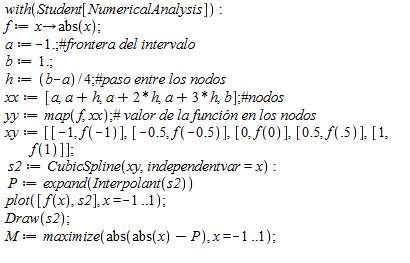But it cannot Find a value for M (wich would be the maximum distance between) it literaly stays working for severeal minutes and necer gives an answer, also i cant find a function to do this for every value for n (n-1 steps from a to b), also would mean that xy would need to get more points, any idea of how can i solve this for any n? or at least fix the problem with M, and i do the steps from a to b Manually.

heeeelp me please this is driving me nuts

thanks a lot :)

## Distance Between 2 curves...

i got 2 curves
a := abs(x);
b := (3/4)*x^2+1/4;

how can i get the max distance between them from x = -1 until x =1?

heeeeeeeeeelp

## Fractional order non linear system...

Hi!

Everyone,

I want to draw  phase plane of system of three fractional order equations.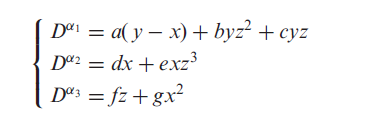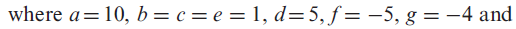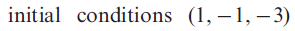Note that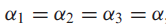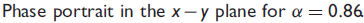Also want the  phase portrait when the values of alpha are not same....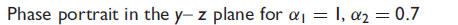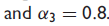Also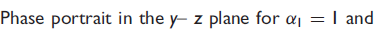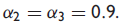Thanks

## My system of equation was ODE, but Maple insists i...

I was trying to solve a system of ODE using Maple, but to my surprise, Maple recognizes diff((phi(t), t)) as a variable which is different than t.

My code is as following:

dsys := {2*m1*(a+l*sin(phi(t)))^2*(diff(diff(theta(t), t), t))+4*m1*(a+l*sin(phi(t)))*l*cos(phi(t))*(diff(theta(t), t))*(diff(phi(t), t)) = M, 2*m1*l^2*(diff(diff(phi(t), t), t))+4*m2*l^2*sin(2*phi(t))*(diff(phi(t), t))*(diff(phi(t), t))+4*m2*l^2*sin(phi(t))^2*(diff(diff(phi(t), t), t))-2*m1*(a+l*sin(phi(t)))*l*cos(phi(t))*(diff(theta(t), t))*(diff(theta(t), t))-2*m2*l^2*(sin(2*phi(t)))(diff(phi(t), t))*(diff(phi(t), t)) = -(2*(m1+m2))*g*l*sin(phi(t))-2*k*l^2*sin(2*phi(t)), phi(0) = 0, theta(0) = 0, (D(phi))(0) = 0, (D(theta))(0) = 0}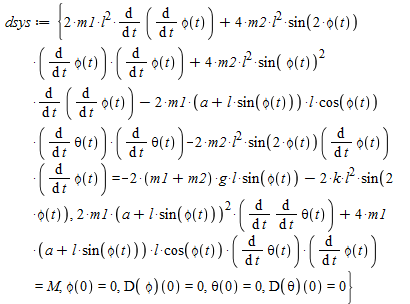subs({M = 10, a = .5, g = 9.81, k = .1, l = .5, m1 = 10, m2 = 1}, dsys);

dsn1 := dsolve(dsys, numeric)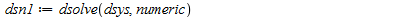The error I got was Error, (in dsolve/numeric/process_input) input system must be an ODE system, got independent variables {t, diff(phi(t), t)}

I don't get why this is happening. Could you show me what's going on?